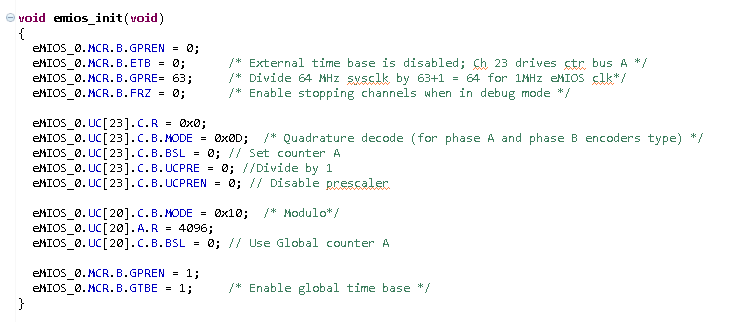# How to setup eMIOS QDEC function?

## How to setup eMIOS QDEC function?

1,136 次查看Contributor III

Hi,

I would like to test the QDEC function of the eMIOS. I am working with MPC5777C on the evaluation board.

I am generating A/B 1khz 90° shift square signals on the EMIOS0/1 channels and I have configured the eMIOS this way :

// IO Pin configuration

emios_configInpuQD = PA_PRIF | IBE;
//eMIOS 0 channel 0
SIU.PCR.R = emios_configInpuQD;
//eMIOS 0 channel 1
SIU.PCR.R = emios_configInpuQD;

//eMIOS configuration

eMIOS_0.MCR.B.GPRE= 63; /* Divide 64 MHz sysclk by 63+1 = 64 for 1MHz eMIOS clk*/
eMIOS_0.MCR.B.ETB = 1; /* External time base is disabled; Ch 23 drives ctr bus A */
eMIOS_0.MCR.B.FRZ = 1; /* Enable stopping channels when in debug mode */

eMIOS_0.UC.C.B.BSL = 3; // Use internal counters?
eMIOS_0.UC.C.B.BSL = 3; // Use internal counters
eMIOS_0.UC.C.B.MODE = 0x0D; /* Quadrature decode (for phase A and phase B encoders type) */

eMIOS_0.MCR.B.GPREN = 1; /* Enable eMIOS clock */
eMIOS_0.MCR.B.GTBE = 1; /* Enable global time base */

I am expecting the see the CNT register of UC(1) incrementing but nothing is happening, looks like I'm missing something very basic. Any clue?

Many thanks,

Daniel

1 解答
867 次查看NXP TechSupport

Hi,

you will need to configure IGF module, otherwise inputs does not pass to eMIOS module.

IGF_0.FILTER.MCR.B.FGEN = 1;

IGF_0.FILTER.MCR.B.FGEN = 1;

BR, Petr

4 回复数
867 次查看Contributor I

Hi,

I would like to test the QDEC function of the eMIOS. I am working with MPC5634 on the evaluation board.

I am generating A/B 1khz 90° shift square signals on the EMIOS8/9 channels and I have configured the eMIOS this way

//MPC5634 - EMIOS -QDEC

void vfn_emios_Qdec_set(uint8_t ui8_chan)
{
EMIOS.CH[ui8_chan-1].CCR.B.MODE= 0x00;
EMIOS.CH[ui8_chan-1].CCNTR.R = 10000;
EMIOS.CH[ui8_chan-1].CBDR.R = 10000;
EMIOS.CH[ui8_chan-1].CCR.B.BSL = 0;
EMIOS.CH[ui8_chan-1].CCR.B.UCPRE = 0;
EMIOS.CH[ui8_chan-1].CCR.B.UCPREN = 1;
EMIOS.CH[ui8_chan-1].CCR.B.EDPOL = 1;

EMIOS.CH[ui8_chan-1].CCR.B.IF= 0x08;
EMIOS.CH[ui8_chan-1].CCR.B.EDSEL=0;
EMIOS.CH[ui8_chan-1].CCR.B.DMA=0;
EMIOS.CH[ui8_chan-1].CCR.B.FEN = 1;

EMIOS.CH[ui8_chan-1].CCR.B.MODE= 0x10;
// EMIOS.CH[ui8_chan-1].CCR.B.MODE= 0x4;

SIU.PCR.R = 0x0500; /* Initialize pad for eMIOS chan. 2 output */

EMIOS.CH[ui8_chan].CCR.B.MODE= 0x00;
EMIOS.CH[ui8_chan].CCNTR.R = 10000;
EMIOS.CH[ui8_chan].CBDR.R = 10000;
EMIOS.CH[ui8_chan].CCR.B.BSL = 0;
EMIOS.CH[ui8_chan].CCR.B.UCPRE = 0;
EMIOS.CH[ui8_chan].CCR.B.UCPREN = 1;
EMIOS.CH[ui8_chan].CCR.B.EDPOL = 1;
;
EMIOS.CH[ui8_chan].CCR.B.IF= 0x08;
EMIOS.CH[ui8_chan].CCR.B.EDSEL=0;
EMIOS.CH[ui8_chan].CCR.B.DMA=0;
EMIOS.CH[ui8_chan].CCR.B.FEN = 1;

EMIOS.CH[ui8_chan].CCR.B.MODE= 0x0D;
// EMIOS.CH[ui8_chan].CCR.B.MODE= 0x02;

SIU.PCR.R = 0x0500; /*4 Initialize pad for eMIOS chan. 2 output */
}

Many thanks,

RH

867 次查看Contributor III

Thanks that was the problem !

Ok now Im trying to drive global counter A with the encoder events.

So I'm configuring UC in QDEC mode and this channel is working fine, I can see the internal counter of UC incrementing correctly but I am not sure if counter A is incrementing

Now I want to configure for example UC with a modulo 4096 driven by counter A, but unfortunatelly this does not work.

Here is my configuration :Regards,

Daniel

867 次查看NXP TechSupport

Hi,

unfortunately counter wrap (e.g. 4095->0 when counting up, 0>4095 when counting down) is not supported by HW for QDEC mode. Neither I do not see SW workaround. You can set CADR register to generate flag on counter match, but this does not bring big benefit.

When ch 23 is running in QDEC mode, its internal counter is providing counter bus A.

If using MC, MCB modes the internal counter is always selected.

BR, Petr

868 次查看NXP TechSupport

Hi,

you will need to configure IGF module, otherwise inputs does not pass to eMIOS module.

IGF_0.FILTER.MCR.B.FGEN = 1;

IGF_0.FILTER.MCR.B.FGEN = 1;

BR, Petr